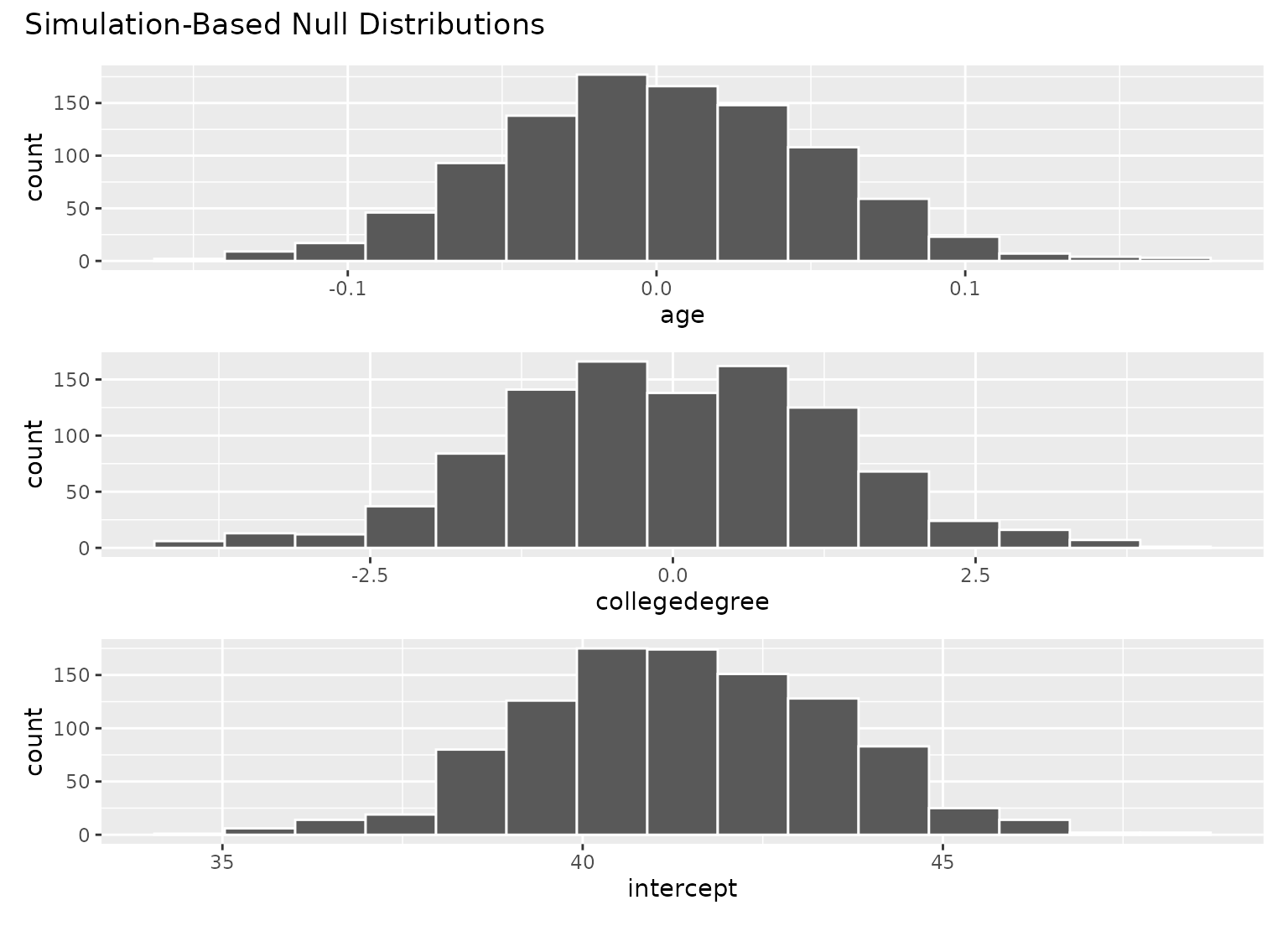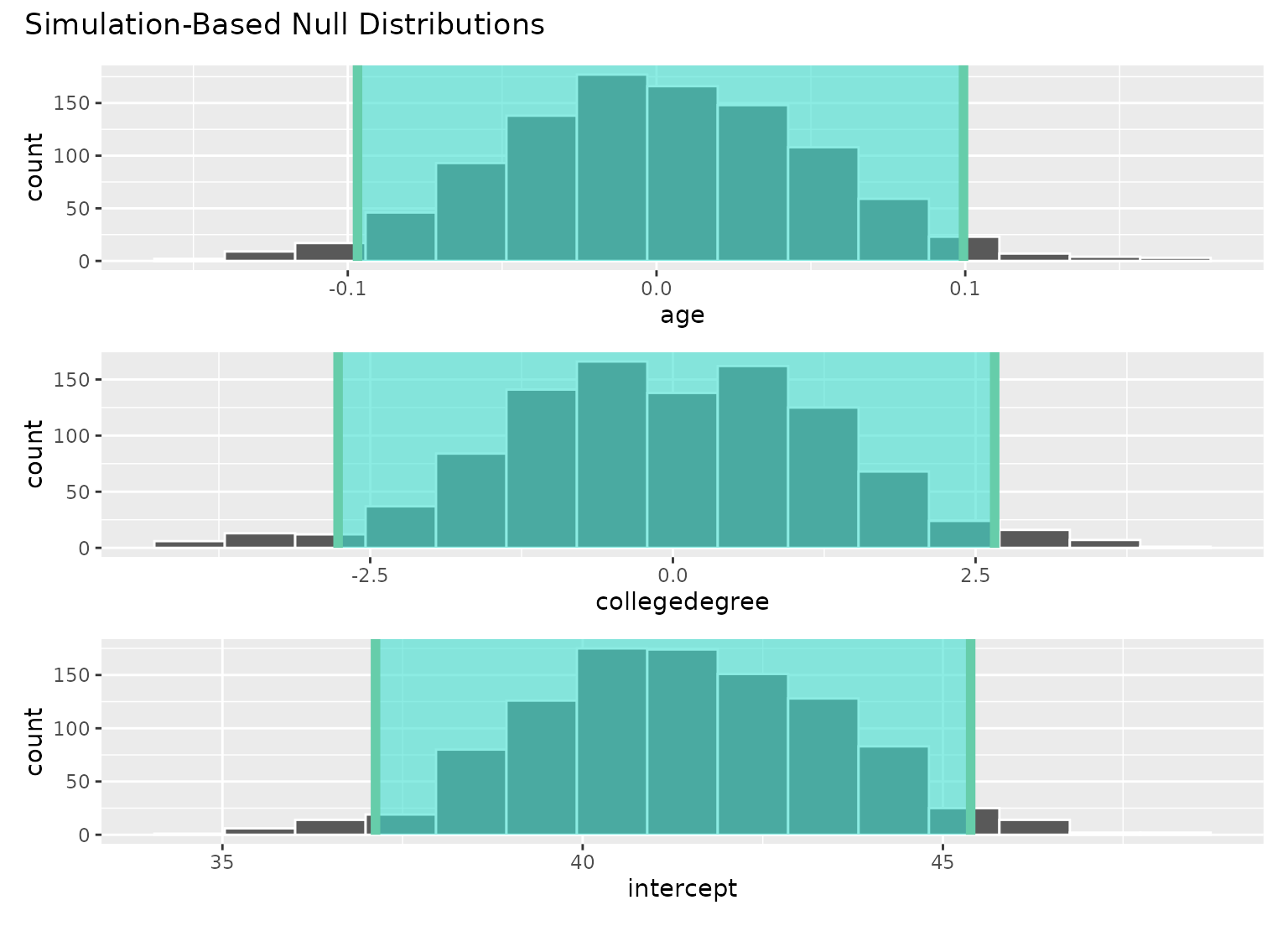shade_confidence_interval() plots a confidence interval region on top of visualize() output. The output is a ggplot2 layer that can be added with +. The function has a shorter alias, shade_ci().

Learn more in vignette("infer").

shade_confidence_interval(
endpoints,
color = "mediumaquamarine",
fill = "turquoise",
...
)

shade_ci(endpoints, color = "mediumaquamarine", fill = "turquoise", ...)

## Arguments

endpoints The lower and upper bounds of the interval to be plotted. Likely, this will be the output of get_confidence_interval(). For calculate()-based workflows, this will be a 2-element vector or a 1 x 2 data frame containing the lower and upper values to be plotted. For fit()-based workflows, a (p + 1) x 3 data frame with columns term, lower_ci, and upper_ci, giving the upper and lower bounds for each regression term. For use in visualizations of assume() output, this must be the output of get_confidence_interval(). A character or hex string specifying the color of the end points as a vertical lines on the plot. A character or hex string specifying the color to shade the confidence interval. If NULL then no shading is actually done. Other arguments passed along to \ggplot2\ functions.

## Value

If added to an existing infer visualization, a \ggplot2\ object displaying the supplied intervals on top of its corresponding distribution. Otherwise, an infer_layer list.

Other visualization functions: shade_p_value()

## Examples

# find the point estimate---mean number of hours worked per week
point_estimate <- gss %>%
specify(response = hours) %>%
calculate(stat = "mean")

# ...and a bootstrap distribution
boot_dist <- gss %>%
# ...we're interested in the number of hours worked per week
specify(response = hours) %>%
# generating data points
generate(reps = 1000, type = "bootstrap") %>%
# finding the distribution from the generated data
calculate(stat = "mean")

# find a confidence interval around the point estimate
ci <- boot_dist %>%
get_confidence_interval(point_estimate = point_estimate,
# at the 95% confidence level
level = .95,
# using the standard error method
type = "se")

# and plot it!
boot_dist %>%
visualize() +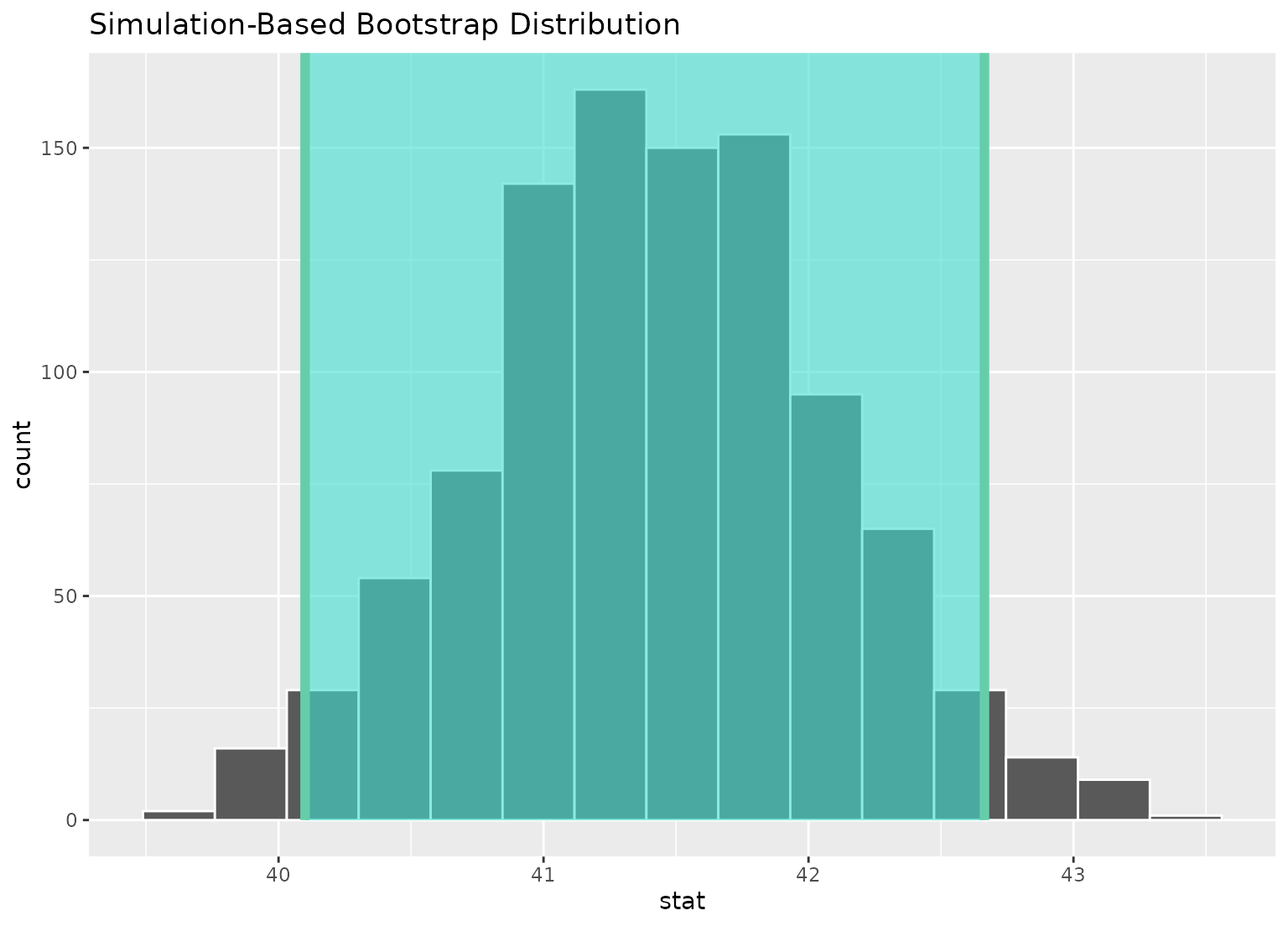# or just plot the bounds
boot_dist %>%
visualize() +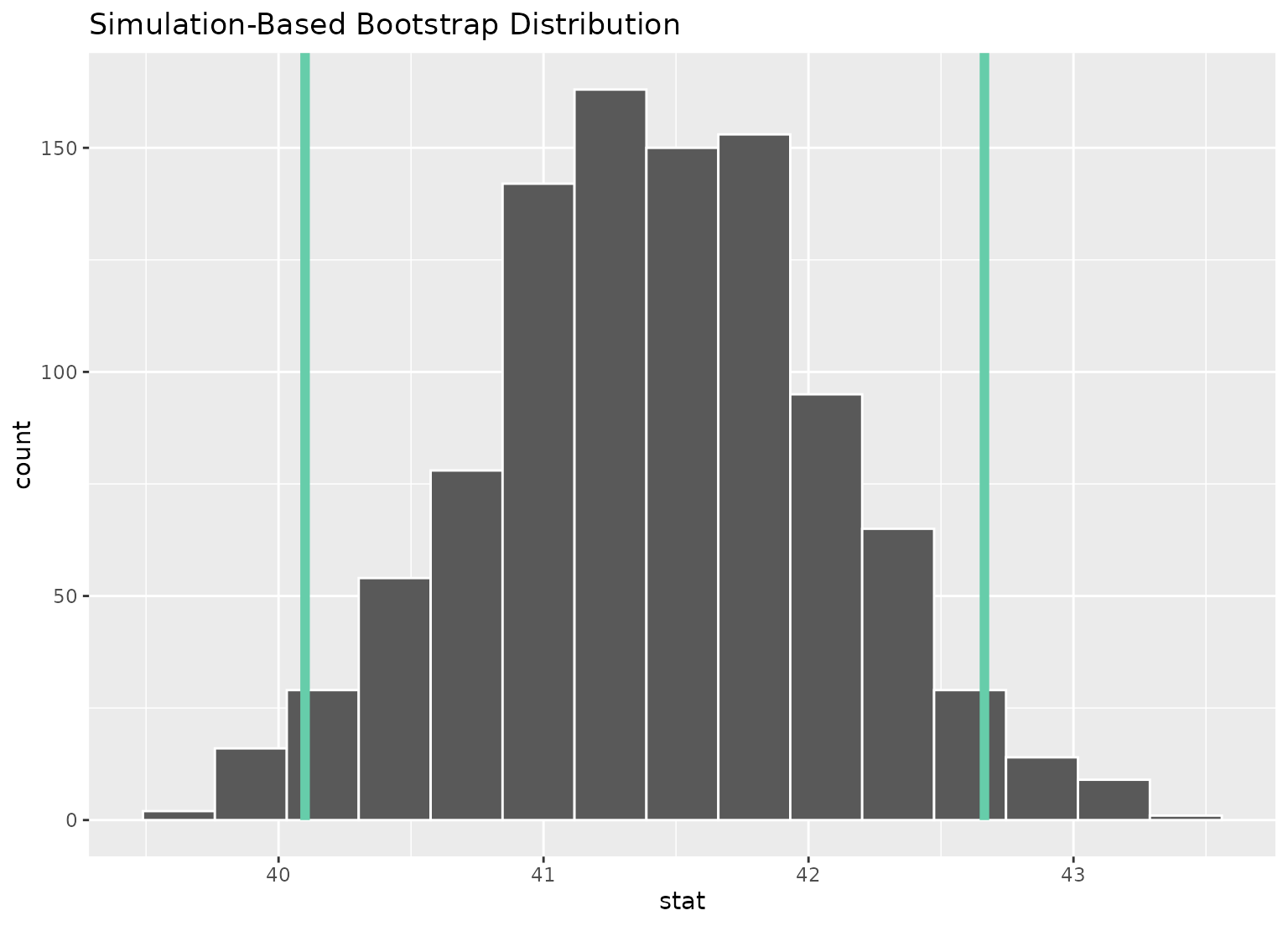# you can shade confidence intervals on top of
# theoretical distributions, too---the theoretical
# distribution will be recentered and rescaled to
# align with the confidence interval
sampling_dist <- gss %>%
specify(response = hours) %>%
assume(distribution = "t")

visualize(sampling_dist) +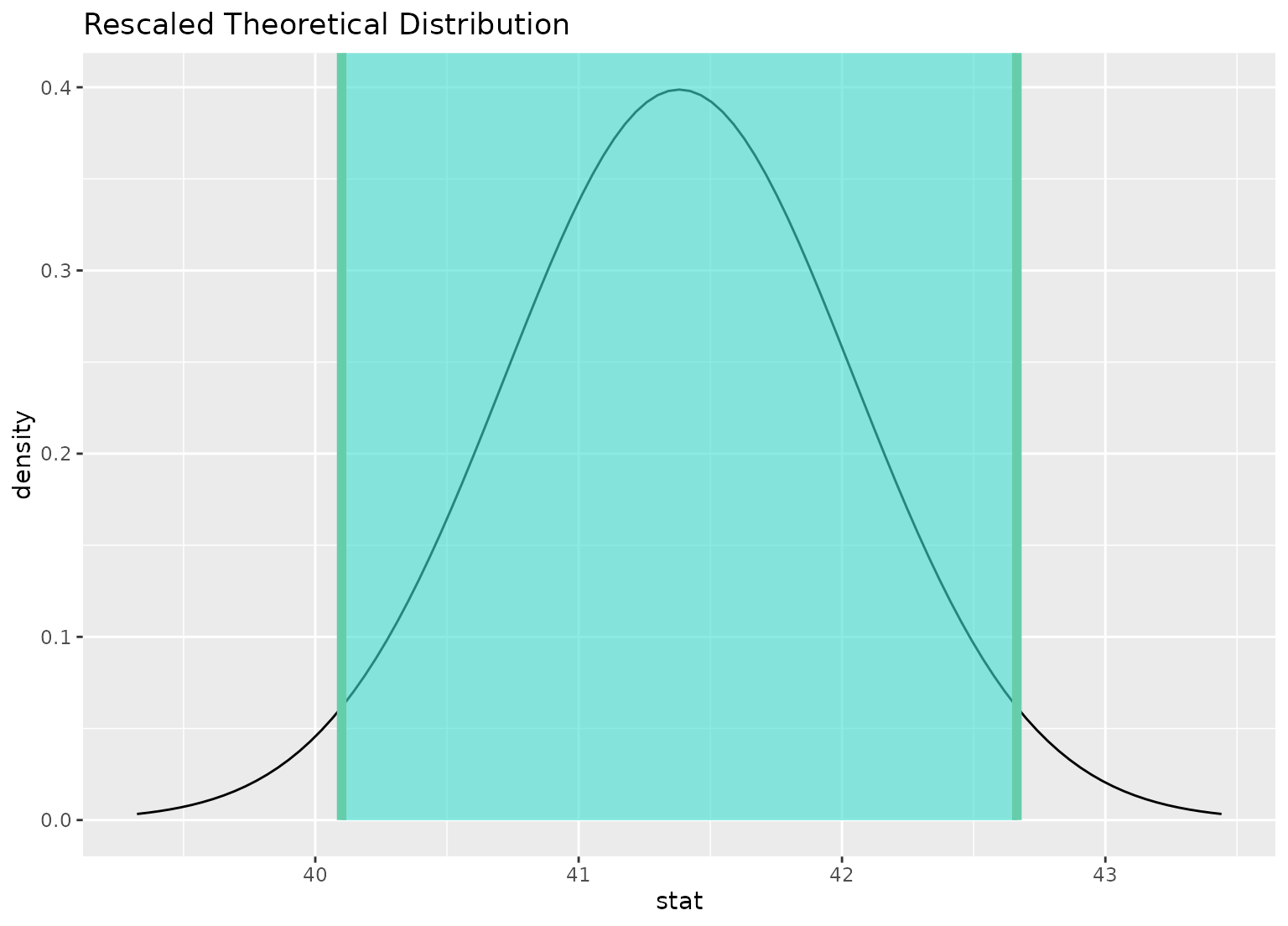# \donttest{
# to visualize distributions of coefficients for multiple
# explanatory variables, use a fit()-based workflow

# fit 1000 linear models with the hours variable permuted
null_fits <- gss %>%
specify(hours ~ age + college) %>%
hypothesize(null = "independence") %>%
generate(reps = 1000, type = "permute") %>%
fit()

null_fits
#> # A tibble: 3,000 × 3
#> # Groups:   replicate [1,000]
#>    replicate term          estimate
#>        <int> <chr>            <dbl>
#>  1         1 intercept      42.9
#>  2         1 age            -0.0507
#>  3         1 collegedegree   1.52
#>  4         2 intercept      40.1
#>  5         2 age             0.0268
#>  6         2 collegedegree   0.632
#>  7         3 intercept      40.0
#>  8         3 age             0.0244
#>  9         3 collegedegree   1.11
#> 10         4 intercept      38.1
#> # … with 2,990 more rows

# fit a linear model to the observed data
obs_fit <- gss %>%
specify(hours ~ age + college) %>%
fit()

obs_fit
#> # A tibble: 3 × 2
#>   term          estimate
#>   <chr>            <dbl>
#> 1 intercept     40.6
#> 2 age            0.00596
#> 3 collegedegree  1.53

# get confidence intervals for each term
conf_ints <-
get_confidence_interval(
null_fits,
point_estimate = obs_fit,
level = .95
)

# visualize distributions of coefficients
# generated under the null
visualize(null_fits)